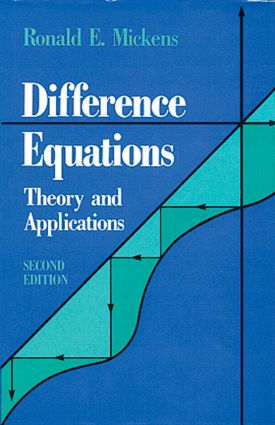Difference Equations, Second Edition

2nd Edition

Chapman and Hall/CRC

464 pages

Purchasing Options:\$ = USD
Hardback: 9780442001360
pub: 1991-01-01
SAVE ~\$36.00
\$180.00
\$144.00
x

FREE Standard Shipping!

Description

In recent years, the study of difference equations has acquired a new significance, due in large part to their use in the formulation and analysis of discrete-time systems, the numerical integration of differential equations by finite-difference schemes, and the study of deterministic chaos. The second edition of Difference Equations: Theory and Applications provides a thorough listing of all major theorems along with proofs. The text treats the case of first-order difference equations in detail, using both analytical and geometrical methods. Both ordinary and partial difference equations are considered, along with a variety of special nonlinear forms for which exact solutions can be determined. Numerous worked examples and problems allow readers to fully understand the material in the text. They also give possible generalization of the theorems and application models.

The text's expanded coverage of application helps readers appreciate the benefits of using difference equations in the modeling and analysis of "realistic" problems from a broad range of fields. The second edition presents, analyzes, and discusses a large number of applications from the mathematical, biological, physical, and social sciences. Discussions on perturbation methods and difference equation models of differential equation models of differential equations represent contributions by the author to the research literature. Reference to original literature show how the elementary models of the book can be extended to more realistic situations.

Difference Equations, Second Edition gives readers a background in discrete mathematics that many workers in science-oriented industries need as part of their general scientific knowledge. With its minimal mathematical background requirements of general algebra and calculus, this unique volume will be used extensively by students and professional in science and technology, in areas such as applied mathematics, control theory, population science, economics, and electronic circuits, especially discrete signal processing.

The Difference Calculus

First-Order Difference Equations

Linear Difference Equations

Linear Difference Equations with Constant Coefficients

Linear Partial Difference Equations

Nonlinear Difference Equations

Problems

Appendix

Notes and References

Bibliography

Index